Background

See Chapter 72, The PRINCOMP Procedure, for a discussion of principal component analysis. See Chapter 26, The CALIS Procedure, for a discussion of confirmatory factor analysis.

Common factor analysis was invented by Spearman (1904). Kim and Mueller (1978a, 1978b) provide a very elementary discussion of the common factor model. Gorsuch (1974) presents a broad survey of factor analysis, and Gorsuch (1974) and Cattell (1978) are useful as guides to practical research methodology. Harman (1976) gives a lucid discussion of many of the more technical aspects of factor analysis, especially oblique rotation. Morrison (1976) and Mardia, Kent, and Bibby (1979) provide excellent statistical treatments of common factor analysis. Mulaik (1972) provides the most thorough and authoritative general reference on factor analysis and is highly recommended to anyone familiar with matrix algebra. Stewart (1981) gives a nontechnical presentation of some issues to consider when deciding whether or not a factor analysis might be appropriate.

A frequent source of confusion in the field of factor analysis is the term factor. It sometimes refers to a hypothetical, unobservable variable, as in the phrase common factor. In this sense, factor analysis must be distinguished from component analysis since a component is an observable linear combination. Factor is also used in the sense of matrix factor, in that one matrix is a factor of a second matrix if the first matrix multiplied by its transpose equals the second matrix. In this sense, factor analysis refers to all methods of data analysis that use matrix factors, including component analysis and common factor analysis.

A common factor is an unobservable, hypothetical variable that contributes to the variance of at least two of the observed variables. The unqualified term "factor" often refers to a common factor. A unique factor is an unobservable, hypothetical variable that contributes to the variance of only one of the observed variables. The model for common factor analysis posits one unique factor for each observed variable.

The equation for the common factor model is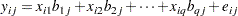where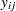is the value of theth observation on theth variable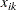is the value of theth observation on theth common factor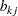is the regression coefficient of theth common factor for predicting theth variable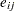is the value of theth observation on theth unique factor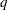is the number of common factors

It is assumed, for convenience, that all variables have a mean of 0. In matrix terms, these equations reduce to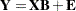In the preceding equation,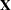is the matrix of factor scores, and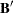is the factor pattern.

There are two critical assumptions:

• The unique factors are uncorrelated with each other.

• The unique factors are uncorrelated with the common factors.

In principal component analysis, the residuals are generally correlated with each other. In common factor analysis, the unique factors play the role of residuals and are defined to be uncorrelated both with each other and with the common factors. Each common factor is assumed to contribute to at least two variables; otherwise, it would be a unique factor.

When the factors are initially extracted, it is also assumed, for convenience, that the common factors are uncorrelated with each other and have unit variance. In this case, the common factor model implies that the covariance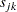between theth andth variables,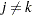, is given by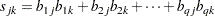or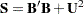where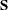is the covariance matrix of the observed variables, and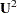is the diagonal covariance matrix of the unique factors.

If the original variables are standardized to unit variance, the preceding formula yields correlations instead of covariances. It is in this sense that common factors explain the correlations among the observed variables. When considering the diagonal elements of standardized, the variance of theth variable is expressed as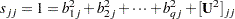where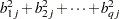and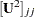are the communality and uniqueness, respectively, of theth variable. The communality represents the extent of the overlap with the common factors. In other words, it is the proportion of variance accounted for by the common factors.

The difference between the correlation predicted by the common factor model and the actual correlation is the residual correlation. A good way to assess the goodness of fit of the common factor model is to examine the residual correlations.

The common factor model implies that the partial correlations among the variables, removing the effects of the common factors, must all be zero. When the common factors are removed, only unique factors, which are by definition uncorrelated, remain.

The assumptions of common factor analysis imply that the common factors are, in general, not linear combinations of the observed variables. In fact, even if the data contain measurements on the entire population of observations, you cannot compute the scores of the observations on the common factors. Although the common factor scores cannot be computed directly, they can be estimated in a variety of ways.

The problem of factor score indeterminacy has led several factor analysts to propose methods yielding components that can be considered approximations to common factors. Since these components are defined as linear combinations, they are computable. The methods include Harris component analysis and image component analysis. The advantage of producing determinate component scores is offset by the fact that, even if the data fit the common factor model perfectly, component methods do not generally recover the correct factor solution. You should not use any type of component analysis if you really want a common factor analysis (Dziuban and Harris; 1973; Lee and Comrey; 1979).

After the factors are estimated, it is necessary to interpret them. Interpretation usually means assigning to each common factor a name that reflects the salience of the factor in predicting each of the observed variables—that is, the coefficients in the pattern matrix corresponding to the factor. Factor interpretation is a subjective process. It can sometimes be made less subjective by rotating the common factors—that is, by applying a nonsingular linear transformation. A rotated pattern matrix in which all the coefficients are close to 0 or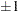is easier to interpret than a pattern with many intermediate elements. Therefore, most rotation methods attempt to optimize a simplicity function of the rotated pattern matrix that measures, in some sense, how close the elements are to 0 or. Because the loading estimates are subject to sampling variability, it is useful to obtain the standard error estimates for the loadings for assessing the uncertainty due to random sampling. Notice that the salience of a factor loading refers to the magnitude of the loading, while statistical significance refers to the statistical evidence against a particular hypothetical value. A loading significantly different from 0 does not automatically mean it must be salient. For example, if salience is defined as a magnitude larger than 0.4 while the entire 95% confidence interval for a loading lies between 0.1 and 0.3, the loading is statistically significant larger than 0 but it is not salient. Under the maximum likelihood method, you can obtain standard errors and confidence intervals for judging the salience of factor loadings.

After the initial factor extraction, the common factors are uncorrelated with each other. If the factors are rotated by an orthogonal transformation, the rotated factors are also uncorrelated. If the factors are rotated by an oblique transformation, the rotated factors become correlated. Oblique rotations often produce more useful patterns than do orthogonal rotations. However, a consequence of correlated factors is that there is no single unambiguous measure of the importance of a factor in explaining a variable. Thus, for oblique rotations, the pattern matrix does not provide all the necessary information for interpreting the factors; you must also examine the factor structure and the reference structure.

Rotating a set of factors does not change the statistical explanatory power of the factors. You cannot say that any rotation is better than any other rotation from a statistical point of view; all rotations, orthogonal or oblique, are equally good statistically. Therefore, the choice among different rotations must be based on nonstatistical grounds. For most applications, the preferred rotation is that which is most easily interpretable, or most compatible with substantive theories.

If two rotations give rise to different interpretations, those two interpretations must not be regarded as conflicting. Rather, they are two different ways of looking at the same thing, two different points of view in the common-factor space. Any conclusion that depends on one and only one rotation being correct is invalid.